Refer to our Texas Go Math Grade 7 Answer Key Pdf to score good marks in the exams. Test yourself by practicing the problems from Texas Go Math Grade 7 Lesson 1.3 Answer Key Adding Rational Numbers.

Reflect

Question 1.
Explain how to determine whether to move right or left on the number line when adding rational numbers.
If the second addend is positive, we move to the right If not, we move to the left.

Use a number line to find each sum.

Question 2.
3 + 1$$\frac{1}{2}$$ = ___________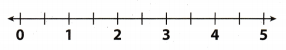Start at 3
Move 1$$\frac{1}{2}$$ units to the right because the second addend is positive
The result is 4.5-2.5 + (-4.5) = ____________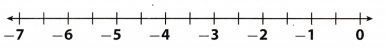Start at 2.5.
Move |-4.5| = |4.5| units to the left because the second addend is negative.
The result is -7.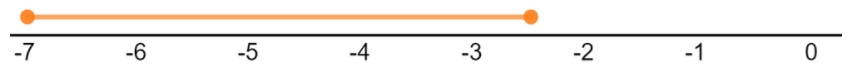Reflect

Question 4.
Do -3 + 2 and 2 + (-3) have the same sum? Does it matter if the negative number is the first addend or the second addend?
Yes, they have the same sum equal to 6. It doesn’t matter since adding is commutative.
(a + b = b + a)

Question 5.
Make a Conjecture Do you think the sum of a negative number and a positive number will always be negative? Explain your reasoning.
No, it will not always be negative.
If the absolute value of a negative number is greater than the absolute value of a positive number, then the sum will be negative.
The sum will be positive ¡n other cases, expect when the absolute values are the same, then the sum will be equal to zero.

Use a number line to find each sum.

Question 6.
-8 + 5 = __________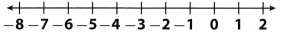Start at -8.
Move 5 units to the right because the second addend is positive
The result is -3.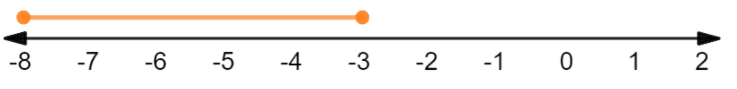Question 7.
$$\frac{1}{2}$$ + (-$$\frac{3}{4}$$) __________Start at $$\frac{1}{2}$$.
Move |-$$\frac{3}{4}$$| = $$\frac{3}{4}$$ units to the left because the second addend ¡s negative.
The result is –$$\frac{1}{4}$$.-1 + 7 = ____________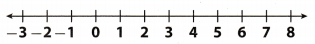Start at -1.
Move 7 units to the right because the second added is positive
The result is 6.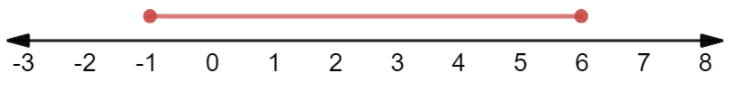Use a number line to find each sum.

Question 9.
2$$\frac{1}{2}$$ + (-2$$\frac{1}{2}$$) = ___________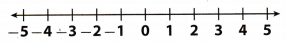Start at 2$$\frac{1}{2}$$.
Move |-2$$\frac{1}{2}$$| = 2$$\frac{1}{2}$$ units to the left because the second addend is negative.
The result is 0.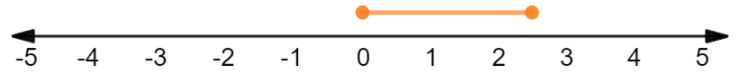Question 10.
-4.5 + 4.5 = _________Start at -4.5.
Move 4.5 units to the right because the second addend is positive.
The result is 0.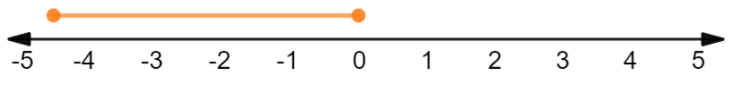Find each sum.

Question 11.
-1.5 + 3.5 + 2 = ____________
Start by grouping numbers with the same sign.
= -1.5 – (3.5 + 2) ………… (1)
= -1.5 – 5.5 …………. (2)
= 4 …………. (3)
(1) Associative property
(2) Add the numbers inside the parentheses.

Question 12.
3$$\frac{1}{4}$$ + (-2) + (-2$$\frac{1}{4}$$) = __________
Start by grouping numbers with the same sign.
= 3$$\frac{1}{4}$$ + ((-2) + (-2$$\frac{1}{4}$$)) …………. (1)
= 3$$\frac{1}{4}$$ + (-4$$\frac{1}{4}$$) ………….. (2)
= -1 …………. (3)
(1) Associative property
(2) Add the numbers inside the parentheses.

Go Math Grade 7 Pdf Algebra Lesson 1.3 Properties Answer Key Question 13.
-2.75 + (-3.25) + 5 = _________
Start by grouping numbers with the same sign.
= (-2.75 + (-3.25)) + 5 …………….. (1)
= -6 + 5 ………… (2)
= -1 ………… (3)
(1) Associative property
(2) Add the numbers inside the parentheses.

Question 14.
15 + 8 + (-3) = ___________
Start by grouping numbers with the same sign.
= (15 + 8) + (-3) ……………. (1)
= 23 + (-3) ………… (2)
= 20 …………… (3)
(1) Associative property
(2) Add the numbers inside the parentheses.

Use a number line to find each sum. (Example 1 and Example 2)

Question 1.
-3 + (-1.5) = ___________Start at -3.
Move |-1.5| = 1.5 units to the left because the second addend is negative.
The result is -4.5.Question 2.
1.5 + 3.5 = ___________Start at 1.5.
Move 3.5 units to the right because the second addend is positive.
The result is 5.Question 3.
$$\frac{1}{4}$$ + $$\frac{1}{2}$$ = ___________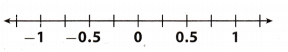Start at $$\frac{1}{4}$$
Move $$\frac{1}{2}$$ units to the right because the second addend is positive.
The result is $$\frac{3}{4}$$.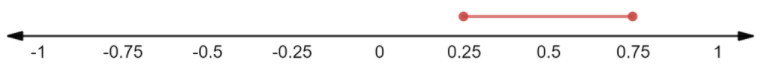Question 4.
-1$$\frac{1}{2}$$ + (-1$$\frac{1}{2}$$) = ____________Start at -1$$\frac{1}{2}$$.
Move |-1$$\frac{1}{2}$$| = 1$$\frac{1}{2}$$ units to the left because the second addend is negative.
The result is -3.3 + (-5) = __________Start at 3.
Move |-5 | = 5 units to the left because the second addend is negative.
The resuLt is -2.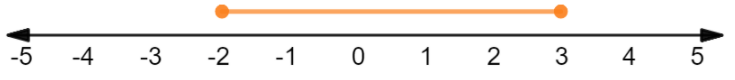Question 6.
-1.5 + 4 = ___________Start at -1.5
Move 4 units to the right because the second addend ¡s posit ive
The result is 2.5.Question 7.
Victor borrowed $21.50 from his mother to go to the theater. A week later, he paid her$21.50 back. How much does he still owe her? (Example 3)
Use a positive number to represent money Victor borrowed from his mother and a negative number to represent money Victor paid back.
Find 21.5 + (-21.5).
Start at 21.5.
Move |-21.5| = 21.5 units to the left because the second addend is negative.
The result is 0. That means he no longer owes her money.Victor owes his mother $0. Question 8. Sandra used her debit card to buy lunch for$8.74 on Monday. On Tuesday, she deposited $8.74 back into her account. What is the overall increase or decrease in her bank account? (Example 3) Answer: We use a positive number to represent the money she deposited into her account, and a negative number to represent the money she spent to buy Lunch. Find -8.74 + 8.74. Start at – 8.74. Move 8.74 units to the right because the second addend is positive. The result is 0. That means there is no increase or decrease.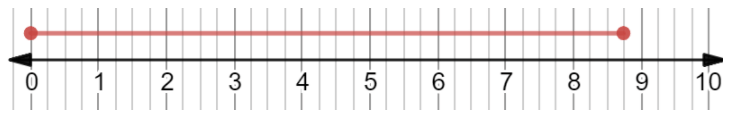There is no increase or decrease. Find each sum without using a number line. (Example 4) Question 9. 2.75 + (-2) + (-5.25) = __________ Answer: Start by grouping numbers with the same sign. = 2.75 + ((-2) + (-5.25)) …………. (1) = 2.75 + (-7.25) …………. (2) = -4.5 ………… (3) (1) Associative property (2) Add the numbers inside the parentheses. Question 10. -3 + (1$$\frac{1}{2}$$) + (2$$\frac{1}{2}$$) = ____________ Answer: Start by grouping numbers with the same sign. = -3+ ((1$$\frac{1}{2}$$) + (2$$\frac{1}{2}$$) ………… (1) = -3 + (4) ………….(2) = 1 …………. (3) (1) Associative property (2) Add the numbers inside the parentheses. Go Math Grade 7 Lesson 1.3 Rational Numbers Question 11. -12.4 + 9.2 + 1 = ___________ Answer: Start by grouping numbers with the same sign. = -12.4 + (9.2 + 1) ………… (1) = -12.4 + (10.2) ………….(2) = -2.2 …………. (3) (1) Associative property (2) Add the numbers inside the parentheses. Question 12. -12 + 8 + 13 = ____________ Answer: Start by grouping numbers with the same sign. = -12 + (8 + 13) ………… (1) = -12 + (21) ………….(2) = 9 …………. (3) (1) Associative property (2) Add the numbers inside the parentheses. Question 13. 4.5 + (-12) + (-4.5) = ____________ Answer: Start by grouping numbers with the same sign. = 4.5 + ((-12) + (-4.5)) ………… (1) = 4.5 + (-16.5) ………….(2) = -12 …………. (3) (1) Associative property (2) Add the numbers inside the parentheses. Question 14. $$\frac{1}{4}$$ + (-$$\frac{3}{4}$$) = __________ Answer: = – $$\frac{2}{4}$$ …………… (1) = – $$\frac{1}{2}$$ …………… (2) (1) Cancel fraction. Question 15. -4$$\frac{1}{2}$$ + 2 = _____________ Answer: = -2$$\frac{1}{2}$$ Question 16. -8 + (-1$$\frac{1}{8}$$) = ___________ Answer: = -9$$\frac{1}{8}$$ Essential Question Check-In Question 17. How can you use a number line to find the sum of -4 and 6? Answer: Start at the -4. Move |6| = 6 units to the right because the second added is positive. The result is 2. Texas Go Math Grade 7 Lesson 1.3 Independent Practice Answer Key Question 18. Samuel walks forward 19 steps. He represents this movement with a positive 19. How would he represent the opposite of this number? Answer: He would represent the opposite of positive 19 with negative 19: -19 Go Math Lesson 1.3 7th Grade Add Fractions Question 19. Julia spends$2.25 on gas for her lawn mower. She earns $15.00 mowing her neighbor’s yard. What is Julia’s profit? Answer: Julia earns$15.00 and spends/Loses $2.25. That means we have to add$15 to her gas expenses of $2.25. -2.25 + 15 = 12.75 Julia’s profit is$12.75

Question 20.
A submarine submerged at a depth of -35.25 meters dives an additional 8.5 meters. What is the new depth of the submarine?
If a submarine is at a depth of -35.25 meters, and dives an additional 8.5 meters, that means we have to add another -8.5 meters to original depth.
-35.25 + (-8.5) = -43.75
New depth of the submarine is -43.75 meters.

Question 21.
Renee hiked for 4$$\frac{3}{4}$$ miles. After resting, Renee hiked back along the same route for 3$$\frac{1}{4}$$ miles. How many more miles does Renee need to hike to return to the place where she started?
We conclude that Renee has to hike 4$$\frac{3}{4}$$ miles back. She already hiked 3$$\frac{1}{4}$$ in opposite direction. To find out how many miles more Renee has to hike, we have to add negative 3$$\frac{1}{4}$$ to 4$$\frac{3}{4}$$.
4$$\frac{3}{4}$$ + (-3$$\frac{1}{4}$$) = 1$$\frac{2}{4}$$
= 1$$\frac{1}{2}$$ miles
(1) Cancel fraction.

Question 22.
Geography The average elevation of the city of New Orleans, Louisiana, is 0.5 m below sea level. The highest point in Louisiana is Driskill Mountain at about 163.5 m higher than New Orleans. How high is Driskill Mountain?
The elevation of New Orleans is -0.5 meters. Driskill Mountain is at 163.5 m higher than New OrLeans To find out how high is Driskitl Mountain, we have to add -0.5 to 163.5.
163.5 + (-0.5) = 163
Driskill mountain is 163 m high.

Question 23.
Problem Solving A contestant on a game show has 30 points. She answers a question correctly to win 15 points. Then she answers a question incorrectly and loses 25 points. What is the contestant’s final score?
If the contestant starts with 30 points, then wins 15 points and Lastly loses 25 points, or wins -25 points, we add 30, 15 and -25 together
30 + 15 + (-25) = 45 + (-25)
= 20
Contestant’s final score is 20 points.

Financial Literacy Use the table for 24-26. Kameh owns a bakery. He recorded the bakery income and expenses in a table.Question 24.
In which months were the expenses greater than the income? Name the month and find how much money was lost.
We can easily Look at the table and find where the expenses are greater than the income.
These months are January and February.
Calculate each month separately by adding expenses and negative income.
January:
1290.60 + (-1205) = 85.60
February:
1345.44 + (-1183) = 162.44

January: $85.60, February:$162.44

Question 25.
In which months was the income greater than the expenses? Name the months and find how much money was gained each of those months.
We can easily Look at the table and find where the income is greater than the expenses.
These months are: June, July, August
Calculate each month separately by adding income and negative expenses.
June: 2413 + (-2106.23) = 309.77
July: 2260 + (-1958.50) = 301.50
August: 2183 + (-1845.12) = 337.88

June: $309.77, July:$301.50, August: $337.88 Question 26. Communicate Mathematical Ideas If the bakery started with an extra$250 from the profits in December, describe how to use the information in the table to figure out the profit or loss of money at the bakery by the end of August. Then calculate the profit or loss.
To determine the profit of these 6 months. we add how much has bakery gained/lost money per month.
-85.60 + (- 162.44) + 0+ 309.77 + 301.50 + 337.88 = 218.01 + 0 + 309.7 + 301.50 – 337.88
= -248.04 + 309.77 + 301.50 + 337.88
= 61.73 + 301.50 + 337.88
= 363.23 + 337.88
= 710.11
Finally, we add the profit from December ($250) and the profit/loss of these 6 months. 250 + 710.11 = 951.11 The bakery has a profit of$951.11.

Question 27.
Vocabulary -2 is the ______________ of 2.
-2 is the opposite of 2.

Question 28.
The basketball coach made up a game to play where each player takes 10 shots at the basket. For every basket made, the player gains 10 points. For every basket missed, the player loses 15 points.
a. The player with the highest score sank 7 baskets and missed 3. What was the highest score?
First, we have to add 10 points 7 times, then add -15 points 3 times.
10 + 10 + 10 + 10 + 10 + 10 + 10 + (-15) + (-15) + (-15) = ………. = 70 + (-35)
= 35

b. The player with the lowest score sank 2 baskets and missed 8. What was the lowest score?
10 + 10 + (-15) + (-15) + (-15) + (-15) + (-15) + (-15) + (-15) + (-15) = ……. = 20 + (-120)
= -100

c. Write an expression using addition to find out what the score would be if a player sank 5 baskets and missed 5 baskets.
First, we have to add 10 points 5 times, then add -15 points 5 times.
10 + 10 + 10 + 10 + 10 + (-15) + (-15) + (-15) + (-15) + (-15) = ….. = 50 + (-105)
= -65

H.O.T.S Focus on Higher Order Thinking

Question 29.
Communicate Mathematical Ideas Explain the different ways it is possible to add two rational numbers and get a negative number.
We can get a negative number if both addends are negative. Also, if only one addend is negative, then its absolute value must be greater than the second addend’s absolute value.

Question 30.
Explain the Error A student evaluated -4 + x for x = -9$$\frac{1}{2}$$ and got the answer of 5$$\frac{1}{2}$$. What might the student have done wrong?
The student might have overseen that the x is negative and calculated as the x was equal to 9$$\frac{1}{2}$$.